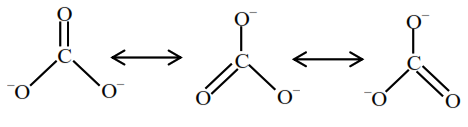# Identify the species having`
Question:

Identify the species having one $\pi$-bond and maximum number of canonical forms from the following:

1. $\mathrm{SO}_{3}$

2. $\mathrm{O}_{2}$

3. $\mathrm{SO}_{2}$

4. $\mathrm{CO}_{3}^{2-}$

Correct Option: , 4

Solution:

Among $\mathrm{SO}_{3}, \mathrm{O}_{2}, \mathrm{SO}_{2}$ and $\mathrm{CO}_{3}^{2-}$, only $\mathrm{O}_{2}$ and $\mathrm{CO}_{3}^{2-}$ has only one $\pi$-bond